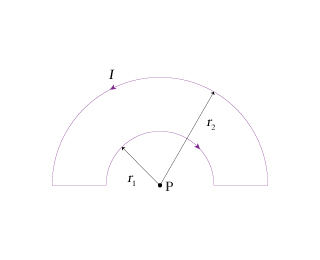# Problem: You may want to review (Page 796).For help with math skills, you may want to review:The Vector Cross Product 1The Vector Cross Product 2What is the strength of the magnetic field at point P in the figure? Assume that I exttip{I}{I}= 5.2 A , r1 exttip{r_{ m 1}}{r_1} = 1.1 cm , and r2 exttip{r_{ m 2}}{r_2} = 2.2 cm .What is the direction of the magnetic field at point P in the figure?

###### FREE Expert Solution

The magnetic field for each semi-circle is given by:

$\overline{){\mathbf{B}}{\mathbf{=}}\frac{{\mathbf{\mu }}_{\mathbf{0}}\mathbf{I}}{\mathbf{4}\mathbf{r}}}$

Let's take the magnetic field on the inner semi-circle to be B1 and that on the outer semicircle to be B2.###### Problem Details

You may want to review (Page 796).

For help with math skills, you may want to review:

The Vector Cross Product 1

The Vector Cross Product 2

What is the strength of the magnetic field at point P in the figure?Assume that I = 5.2 A , r1 = 1.1 cm , and r2 = 2.2 cm .

What is the direction of the magnetic field at point P in the figure?